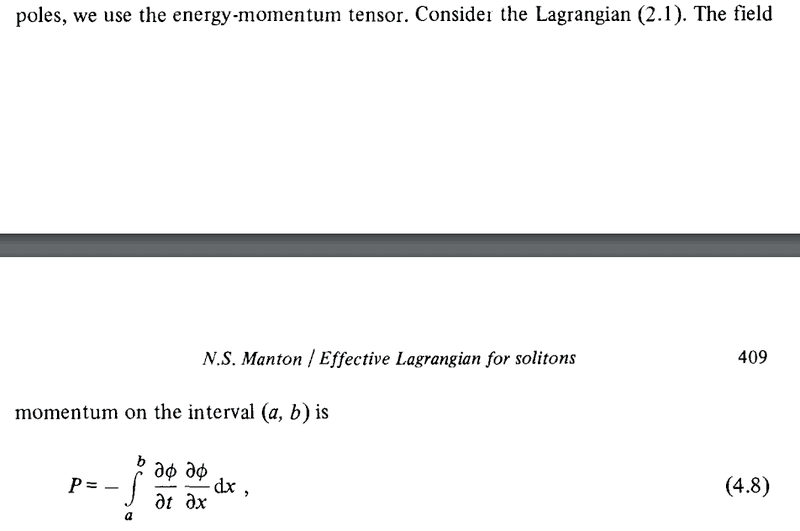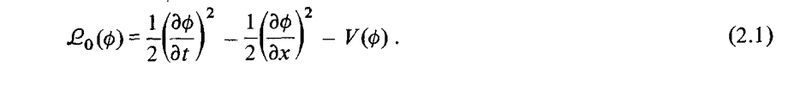# One-dimensional field momentum

How does one arrive at the formula 4.8?The Lagrangian (one spatial dimension) is:vanhees71
$$\Theta^{\mu \nu}=\frac{\partial \mathcal{L}}{\partial (\partial_{\nu} \phi)}\partial^{\mu} \phi-\mathcal{L} g^{\mu \nu}.$$
•